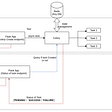# Generator and Traversal

`-- |It creates a list of integers from 1 to infinitylist = [1..]take 10 list-- | Prints out first 10 digits from the list=> [1,2,3,4,5,6,7,8,9,10]`
`def sample_generator_function():    print('Before yield')    yield    print('After yield')`
`# calling the generator function will just return you a generator object unlike the normal function.generator_func = sample_generator_function()# now we need to pass this generator object to a built-in function called nextnext(generator_func)#this will print 'Before yield'# since we hit the yield statement, generator function is suspended# in order to execute later statements, we need to again call next on itnext(generator_func)#this will print 'After yield'`
`def infinite_series():    a = 0    while True:        yield a        a += 1# start the generatorgen = infinite_series()print(next(gen)) # 0print(next(gen)) # 1print(next(gen)) # 2# this looks way too much of work, however there are standard library in rescue to do just thatfrom itertools import isliceprint(list(islice(gen, 3)))# [3, 4, 5]print(list(islice(gen, 3)))# [6, 7, 8]`
`function* fib() {  let a = 0;  let b = 1;  while (true) {    yield a;    temp = a;    a = b;    b = temp + b;  }}// Let's execute it manuallyconst fibGenerator = fib();const firstValue = fibGenerator.next().valueconst secondValue = fibGenerator.next().valueconst thirdValue = fibGenerator.next().value// lets write a utility function to abstract out the manual executionfunction take(n, gen) {  const result = [];  while(n--) {    const val = gen.next().value;    result.push(val);  }  return result;}// now we can write something like thisconst series = take(5, fibGenerator);console.log(series) // [2, 3, 5, 8, 13]`
`''' Generator function that yields a value from 0 to < n'''def range(n):    i = 0    while i < n:        yield i        i += 1`
`for val in range(5):    print(val, end=' ')# output# 0 1 2 3 4`
`def my_another_generator():    for i in range(5):        yield i    yield 'done'`
`for val in my_another_generator():    print(val, end=' ')# output# 0 1 2 3 4 'done'`
`def my_another_generator():    yield from range(5)  # notice the "from" keyword    yield 'done'for val in my_another_generator():    print(val, end=' ')# output# 0 1 2 3 4 'done'`
`def ping_pong():    yield 'ping'    yield 'pong'    yield from ping_pong()# lets first make a generator objectgen = ping_pong()# lets drive thisnext(gen) # "ping"next(gen) # "pong"next(gen) # "ping"next(gen) # "pong"next(gen) # "ping"next(gen) # "pong"# it goes on forever `
`'''Fibonacci series using recursive generator delegation'''def fib(a, b):   yield a   yield from fib(b, a + b)`
`class Node:    def __init__(self, val, next_node=None):        self.val = val        self.next_node = next_node    def __repr__(self):        return f'Node({self.val})'# function to convert normal list to linked listdef from_list(alist):    if len(alist) == 1:        return Node(alist)    return Node(alist, next_node=from_list(alist[1:]))alist = [4, 5, 6, 7, 8]#converting to linked listlinked_list = from_list(alist)`
`def traverse(node):    if node:        yield node        yield from traverse(node.next_node)`
`def traverse_tree(node):    if node:        yield from traverse_tree(node.left)        yield node        yield from traverse_tree(node.right)`

--

--

--

## More from Robus Gauli

https://github.com/RobusGauli

Love podcasts or audiobooks? Learn on the go with our new app.

## [rmatz@localhost ~] whoami## ScyllaDB and CASSANDRA BASED FULL HISTORY SOLUTION FOR EOS ECOSYSTEM## An SQL Editor for Apache Spark SQL with Livy## Playing with Prometheus…## Introduction to Analysis and analyzers in Elasticsearch## The good & the bad side of being a girl developer.## Plena Integrates With Transak## Robus Gauli

https://github.com/RobusGauli

## More from Medium